### Home > CALC > Chapter 1 > Lesson 1.1.1 > Problem1-9

1-9.
1. Now you know two general equations used to write the equation of a line:

y = mx + b and y = m(xh) + k
Under what circumstances is each equation easier to use? For parts (a) through (c) below, determine which method is best with the given information. Then, write the equation for the line. Homework Help ✎

1.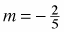and through (−6, 2)

2. m = 3 and b = −6

3. Through (2, 8) and (1, 3).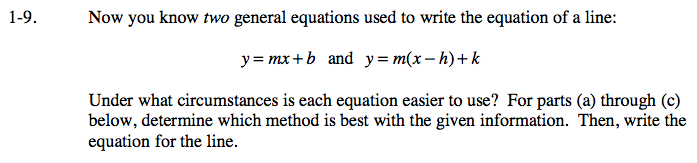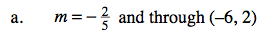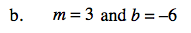'm' stands for slope.
'b' stands for y-intercept.
What information is given?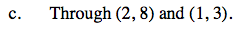To find the slope between two points, use:

$m=\frac{y_2-y_1}{x_2-x_1}$